×#### Thank you for registering.

One of our academic counsellors will contact you within 1 working day.

Click to Chat

1800-1023-196

+91-120-4616500

CART 0

• 0

MY CART (5)

Use Coupon: CART20 and get 20% off on all online Study Material

ITEM
DETAILS
MRP
DISCOUNT
FINAL PRICE
Total Price: Rs.

There are no items in this cart.
Continue Shopping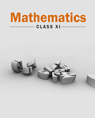• Complete Mathematics Course - Class 11
• OFFERED PRICE: Rs. 4,240
• View Details

```IIT JEE Mathematics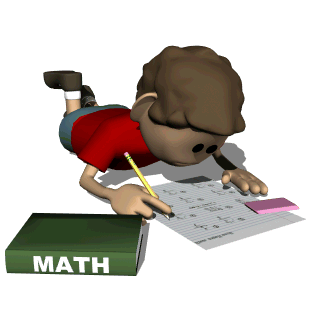Mathematics is the most vital part of the IIT JEE syllabus. It is in fact an indispensible part of human race. Physics, Chemistry and Mathematics have equal weightage in the JEE but Mathematics has an edge over the other two as, in case of a tie in the overall score of two or more candidates, the score in mathematics is considered to be the deciding factor. Mathematics is like a kin of science which lays the foundation for Physics and Chemistry. Various topics like calculus, differential equations and quadratic equations are used in almost all the questions of physics and chemistry.As a matter of fact, Science progresses only with the aid of Mathematics. It is aptly remarked that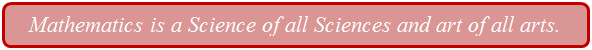The syllabus of IIT JEE Mathematics is a perfect blend of easy and difficult topics. Topics like probability, differential calculus, trigonometry, straight lines and circles in coordinate geometry, permutations and combinations in algebra are always easy to crack in IIT JEE. The success mantra of the JEE is practice and hard work. Gone are the days when students used to spend hours in attempting one question. Now it is an era of multiple choice questions. The JEE Mathematics questions test a student’s acquired knowledge as well as his aptitude.There are various mathematics books for IIT JEE which explain all the important topics in detail. IIT JEE Math is not very tough but students fail to excel in it as their basics are not very clear.  Mathematics is a creation of human mind that builds up the reasoning capability in humans. It is this branch which explains the logic behind the concepts. Mathematical concepts lay down the foundation for Physics as well as Chemistry. The topics which form a part of IIT mathematics syllabus include: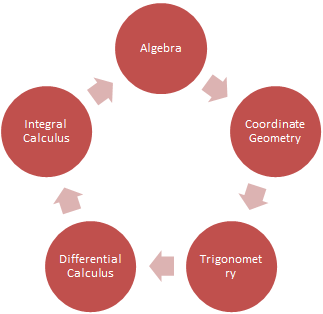All these domains are equally important and can be mastered with consistent practice and hard work. We discuss all of them one by one:

Algebra:

Algebra can be considered to be one of the simplest and the most scoring domain of IIT Mathematics. The topics are quite interesting and demand good computation speed. In algebra, it is important to have conceptual clarity along with good speed in order to fetch excellent scores in various competitive exams like the JEE. Some of the important areas covered under it include:Sets, Relations & Functions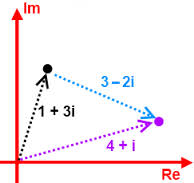Complex Numbers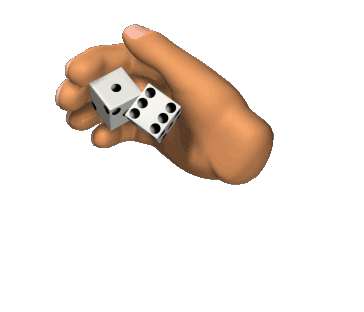Probability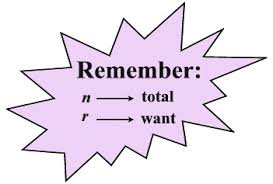Permutations & CombinationsMatrices & Determinants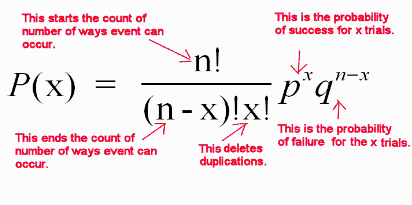Binomial Theorem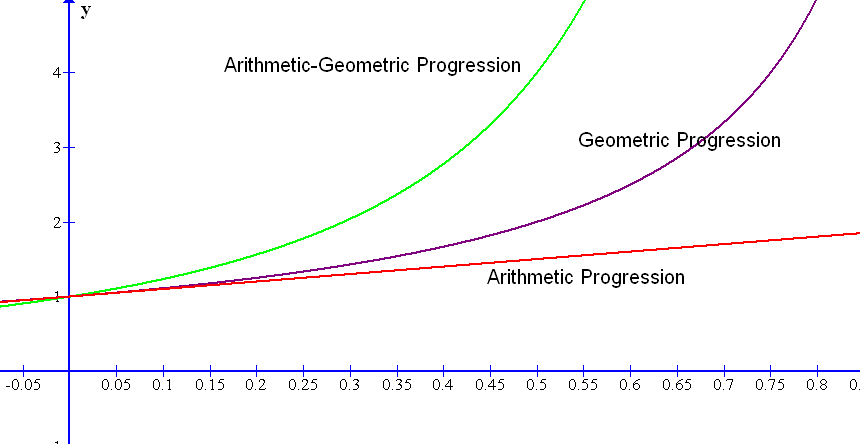Progressions & Series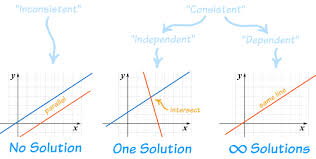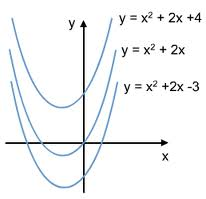Differential Equations

Differential Calculus & Integral Calculus:

Calculus is the most important topic for JEE (Main & Advanced) and it constitutes a major part of modern mathematics. It has two major branches, differential calculus and integral calculus, which are related by the fundamental theorem of calculus. Calculus is the study of change, in the same way that geometry is the study of shape and algebra is the study of operations and their application to solving equations. The JEE Math comprises all sort of problems including the theoretical as well as application based.

Differential Calculus: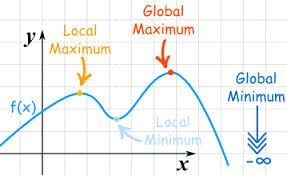Apllication of Derivatives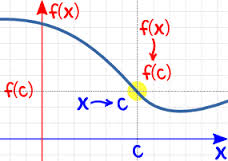Limits & Continuity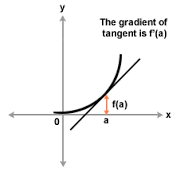Differentiation

Integral Calculus: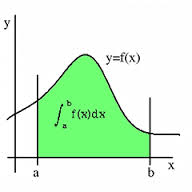Definite Integral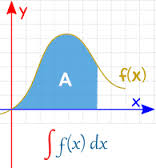Indefinite Integral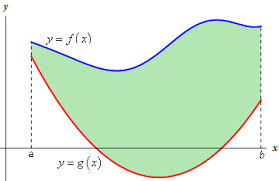Area under Curves

Coordinate Geometry:

Coordinate geometry is also an important component of IIT Math. It is basically a method of analyzing geometrical shapes and is one of the most scoring topics of the mathematics syllabus of IIT JEE and other engineering exams. Besides calculus, this is the only topic that can fetch you maximum marks. It is a vast topic and can further be divided into various parts like: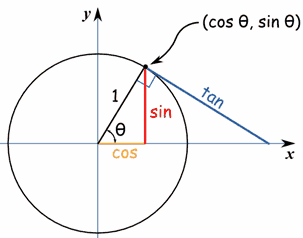CircleParabola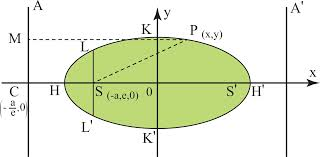Ellipse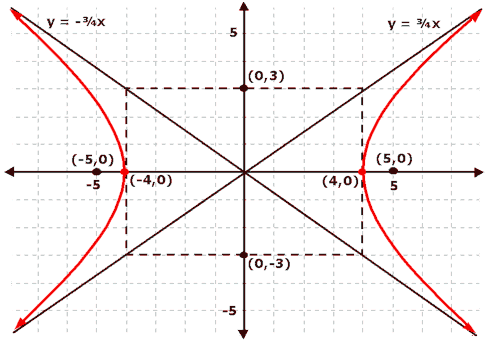Hyperbola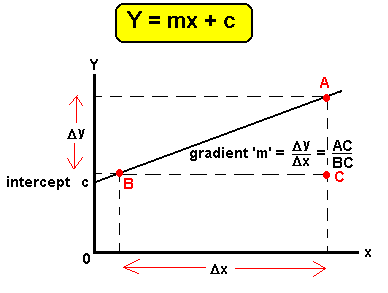Straight Lines

Majority of the mathematics questions are often framed from the topics of Straight Line and the Circle. These topics together fetch maximum questions in the JEE and they are a pre requisite to conic sections as well.Trigonometry:

Trigonometry is a vital constituent of Mathematics in IIT JEE examination. Trigonometric functions and trigonometry ratios are some of the most imperative areas of trigonometry. Since the IIT JEE exam asks a good amount of questions on this topic, so getting the knack of the basic trigonometry can surely help an IIT JEE aspirant to smooth his way through the exam.

This branch of maths deals with triangles and the relationships between the sides and angles. The trigonometry functions are universal in parts of pure mathematics and applied mathematics which also lay the groundwork for many branches of science and technology. The IIT JEE Trigonometry problems range from the trigonometry basics to the applications of trigonometry.

If one prepares the topics efficiently, he can easily get a good AIR. So if you have practiced all types of IIT maths questions thoroughly it would help you in reaching the solution quickly rather than figuring out how to crack the question. The more one practices and learns the typical methods of solving different types of questions the faster he can crack IIT JEE mathematics questions. A person should also try to apply various IIT Maths tricks to come out with flying colors.

Just change your approach towards mathematics and you’ll realize how lively this subject is!

```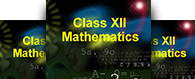### Course Features

• 90 Video Lectures
• Revision Notes
• Previous Year Papers
• Mind Map
• Study Planner
• NCERT Solutions
• Discussion Forum
• Test paper with Video Solution